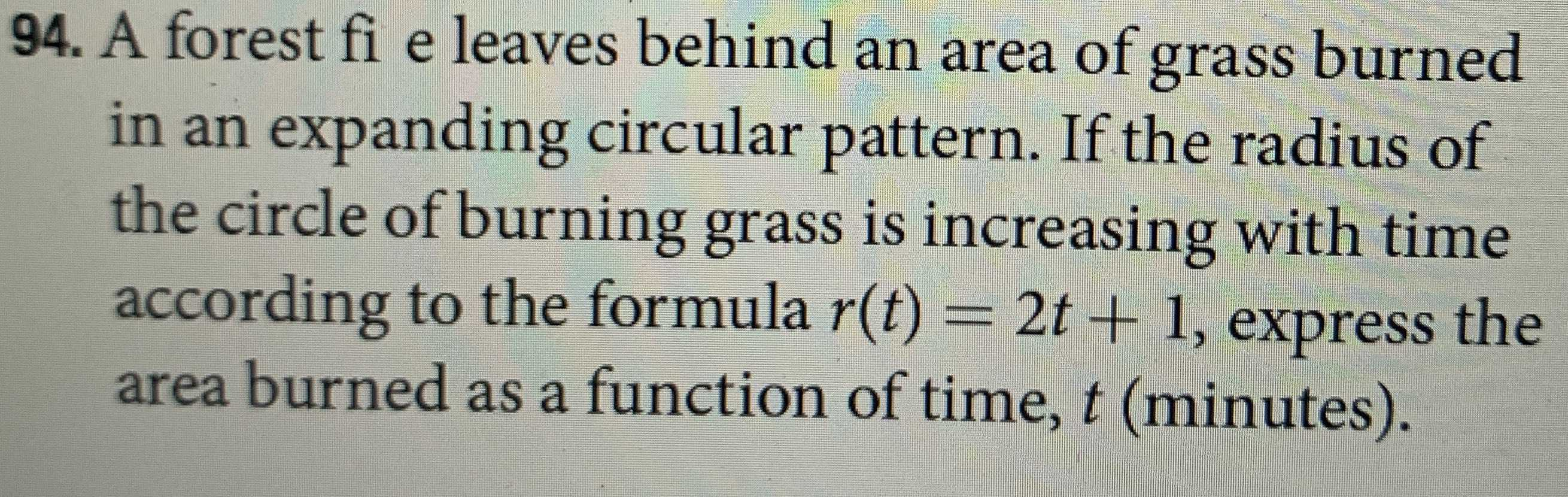### Still have math questions?94. A forest fi e leaves behind an area of grass burned in an expanding circular pattern. If the radius of the circle of burning grass is increasing with time according to the formula $$r ( t ) = 2 t + 1$$ , express the area burned as a function of time, $$t$$ (minutes).
$$(4t^{2}+ 4t+ 1)\pi$$